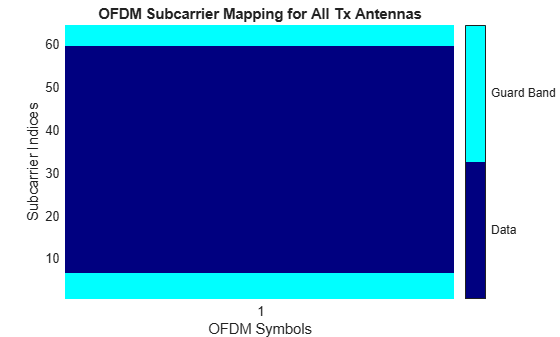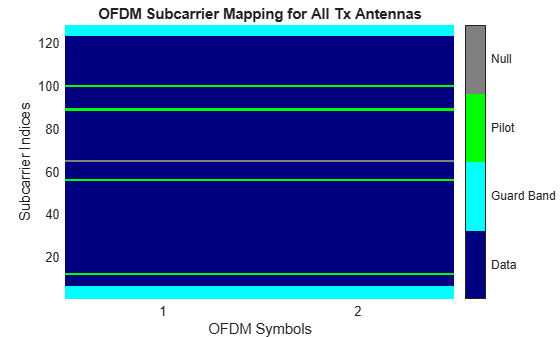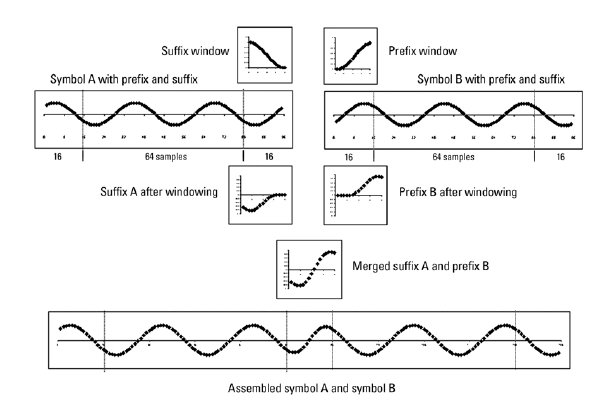Documentation

comm.OFDMModulator

Modulate using OFDM method

Description

The OFDMModulator object modulates using the orthogonal frequency division modulation method. The output is a baseband representation of the modulated signal.

To modulate an OFDM signal:

1. Define and set up the OFDM modulator object. See Construction.

2. Call step to modulate a signal according to the properties of comm.OFDMModulator. The behavior of step is specific to each object in the toolbox.

Note

Starting in R2016b, instead of using the step method to perform the operation defined by the System object™, you can call the object with arguments, as if it were a function. For example, y = step(obj,x) and y = obj(x) perform equivalent operations.

Construction

H = comm.OFDMModulator creates a modulator System object, H, that modulates the input signal using the orthogonal frequency division modulation (OFDM) method.

H = comm.OFDMModulator(Name,Value) creates a OFDM modulator object, H, with each specified property set to the specified value. You can specify additional name-value pair arguments in any order as (Name1,Value1,...,NameN,ValueN).

H = comm.OFDMModulator(hDemod) creates an OFDM modulator object, H, whose properties are determined by the corresponding OFDM demodulator object, hDemod.

Properties

 FFTLength The length of the FFT, NFFT, is equivalent to the number of subcarriers used in the modulation process. FFTLength must be ≥ 8. Specify the number of subcarriers. The default is 64. NumGuardBandCarriers The number of guard band subcarriers allocated to the left and right guard bands. Specify the number of left and right subcarriers as nonnegative integers from 0 to (floor(FFTLength / 2 ) − 1) where you specify the left, NleftG, and right, NrightG, guard bands independently in a 2-by-1 column vector. The default values are [6; 5]. InsertDCNull This is a logical variable that controls whether a DC null is inserted. The default value is false. The DC subcarrier is the center of the frequency band and has the index value: (FFTLength / 2) + 1 when FFTLength is even(FFTLength + 1) / 2 when FFTLength is odd PilotInputPort This is a logical property that controls whether you can specify the pilot carrier indices. If true, you can assign individual subcarriers for pilot transmission; otherwise, pilot information will be assumed to be embedded in the input data. The default value is false. PilotCarrierIndices If the comm.OFDMModulator.PilotInputPort property is set to true, you can specify the indices of the pilot subcarriers. You can assign the indices to the same or different subcarriers for each symbol. Similarly, the pilot carrier indices can differ across multiple transmit antennas. Depending on the desired level of control for index assignments, the dimensions of the property vary. Valid pilot indices fall in the range $\left[{\text{N}}_{\text{leftG}}+1,\text{\hspace{0.17em}}{\text{N}}_{\text{FFT}}/2\right]\cup \left[{\text{N}}_{\text{FFT}}/2+2,\text{\hspace{0.17em}}{\text{N}}_{\text{FFT}}-{\text{N}}_{\text{rightG}}\right],$where the index value cannot exceed the number of subcarriers. When the pilot indices are the same for every symbol and transmit antenna, the property has dimensions Npilot-by-1, where Npilot is the number of pilot subcarriers. When the pilot indices vary across symbols, the property has dimensions of Npilot-by-Nsym, where Nsym is the number of symbols. If there is only one symbol but multiple transmit antennas, the property has dimensions of Npilot-by-1-by-NT, where NT is the number of transmit antennas. If the indices vary across the number of symbols and transmit antennas, the property has dimensions of Npilot-by-Nsym-by-NT. It is desirable that when the number of transmit antennas is greater than one, the indices per symbol should be mutually distinct across antennas to avoid interference. The default value is [12; 26; 40; 54]. CyclicPrefixLength The CyclicPrefixLength property specifies the length of the OFDM cyclic prefix. If you specify a scalar, the prefix length is the same for all symbols through all antennas. If you specify a row vector of length Nsym, the prefix length can vary across symbols but remains the same length through all antennas. The default value is 16. Windowing This is a logical property whose state enables or disables windowing. Windowing is the process in which the OFDM symbol is multiplied by a raised cosine window before transmission to more quickly reduce the power of out-of-band subcarriers. This serves to reduce spectral regrowth. The default value is false. WindowLength This property specifies the length of the raised cosine window when comm.OFDMModulator.Windowing is true. Use positive integers with a maximum value no greater than the minimum cyclic prefix length. For example, in a configuration having four symbols with cyclic prefix lengths of [12 16 14 18], the window length cannot exceed 12. The default value is 1. NumSymbols This property specifies the number of symbols, Nsym. NumSymbols must be a positive integer. The default value is 1. NumTransmitAntennnas This property determines the number of antennas, NT, used to transmit the OFDM modulated signal. The property is a positive integer. The default value is 1.

Methods

 info Provide dimensioning information for the OFDM method reset Reset states of the OFDMModulator System object showResourceMapping Show the subcarrier mapping of the OFDM symbols created by the OFDM modulator System object. step Modulate using OFDM method
Common to All System Objects
release

Allow System object property value changes

Examples

expand all

An OFDM modulator System object™ can be constructed using default properties. Once constructed, these properties can be modified.

Construct an OFDM modulator.

ofdmMod = comm.OFDMModulator;

Display the properties of the modulator.

disp(ofdmMod)
comm.OFDMModulator with properties:

FFTLength: 64
NumGuardBandCarriers: [2x1 double]
InsertDCNull: false
PilotInputPort: false
CyclicPrefixLength: 16
Windowing: false
NumSymbols: 1
NumTransmitAntennas: 1

Modify the number of subcarriers and symbols.

ofdmMod.FFTLength = 128;
ofdmMod.NumSymbols = 2;

Verify that the number of subcarriers and the number of symbols changed.

disp(ofdmMod)
comm.OFDMModulator with properties:

FFTLength: 128
NumGuardBandCarriers: [2x1 double]
InsertDCNull: false
PilotInputPort: false
CyclicPrefixLength: 16
Windowing: false
NumSymbols: 2
NumTransmitAntennas: 1

The showResourceMapping method shows the mapping of data, pilot, and null subcarriers in the time-frequency space. Apply the showResourceMapping method.

showResourceMapping(ofdmMod)An OFDM modulator System object™ can be constructed from an existing OFDM demodulator System object.

Construct an OFDM demodulator, ofdmDemod and specify pilot indices for a single symbol and two transmit antennas.

Note: You can set the PilotCarrierIndices property in the demodulator object, which then changes the number of transmit antennas in the modulator object. The number of receive antennas in the demodulator is uncorrelated with the number of transmit antennas.

ofdmDemod = comm.OFDMDemodulator;
ofdmDemod.PilotOutputPort = true;
ofdmDemod.PilotCarrierIndices = cat(3,[12; 26; 40; 54],...
[13; 27; 41; 55]);

Use the demodulator, ofdmDemod, to construct the OFDM modulator.

ofdmMod = comm.OFDMModulator(ofdmDemod);

Display the properties of the modulator and verify that they match those of the demodulator.

disp(ofdmMod)
comm.OFDMModulator with properties:

FFTLength: 64
NumGuardBandCarriers: [2x1 double]
InsertDCNull: false
PilotInputPort: true
PilotCarrierIndices: [4x1x2 double]
CyclicPrefixLength: 16
Windowing: false
NumSymbols: 1
NumTransmitAntennas: 2
disp(ofdmDemod)
comm.OFDMDemodulator with properties:

FFTLength: 64
NumGuardBandCarriers: [2x1 double]
RemoveDCCarrier: false
PilotOutputPort: true
PilotCarrierIndices: [4x1x2 double]
CyclicPrefixLength: 16
NumSymbols: 1

The showResourceMapping method displays the time-frequency resource mapping for each transmit antenna.

Construct an OFDM modulator.

mod = comm.OFDMModulator;

Apply the showResourceMapping method.

showResourceMapping(mod)Insert a DC null.

mod.InsertDCNull = true;

Show the resource mapping after adding the DC null.

showResourceMapping(mod)The OFDM modulator enables you to specify the subcarrier indices for the pilot signals. The indices can be specified for each symbol and transmit antenna. When there is more than one transmit antenna, ensure that the pilot indices for each symbol differ between antennas.

Construct an OFDM modulator that has two symbols and insert a DC null.

mod = comm.OFDMModulator('FFTLength',128,'NumSymbols',2,...
'InsertDCNull',true);

Turn on the pilot input port so you can specify the pilot indices.

mod.PilotInputPort = true;

Specify the same pilot indices for both symbols.

mod.PilotCarrierIndices = [12; 56; 89; 100];

Visualize the placement of the pilot signals and nulls in the OFDM time-frequency grid using the showResourceMapping method.

showResourceMapping(mod)Concatenate a second column of pilot indices to the PilotCarrierIndices property to specify different indices for the second symbol.

mod.PilotCarrierIndices = cat(2, mod.PilotCarrierIndices, ...
[17; 61; 94; 105]);

Verify that the pilot subcarrier indices differ between symbols.

showResourceMapping(mod)Increase the number of transmit antennas to two.

mod.NumTransmitAntennas = 2;

Specify the pilot indices for each of the two transmit antennas. To provide indices for multiple antennas while minimizing interference among the antennas, populate the PilotCarrierIndices property as a 3-D array such that the indices for each symbol differ among antennas.

mod.PilotCarrierIndices = cat(3,[20; 50; 70; 110], ...
[15; 60; 75; 105]);

Display the resource mapping for the two transmit antennas. The gray lines denote the insertion of custom nulls. The nulls are created by the object to minimize interference among the pilot symbols from different antennas.

showResourceMapping(mod)Specify the length of the cyclic prefix for each OFDM symbol.

Construct an OFDM modulator having five symbols, four left guard-band subcarriers, and three right guard-band subcarriers. Specify the cyclic prefix length for each OFDM symbol.

mod = comm.OFDMModulator('NumGuardBandCarriers',[4;3],...
'NumSymbols',5,...
'CyclicPrefixLength',[12 10 14 11 13]);

Display the properties of the modulator and verify that the cyclic prefix length changes across symbols.

disp(mod)
comm.OFDMModulator with properties:

FFTLength: 64
NumGuardBandCarriers: [2x1 double]
InsertDCNull: false
PilotInputPort: false
CyclicPrefixLength: [12 10 14 11 13]
Windowing: false
NumSymbols: 5
NumTransmitAntennas: 1

Determine the OFDM modulator data dimensions by using the info method.

Construct an OFDM modulator System object™ with user-specified pilot indices, insert a DC null, and specify two transmit antennas.

hMod = comm.OFDMModulator('NumGuardBandCarriers',[4;3], ...
'PilotInputPort',true, ...
'PilotCarrierIndices',cat(3,[12; 26; 40; 54], ...
[11; 25; 39; 53]), ...
'InsertDCNull',true, ...
'NumTransmitAntennas',2);

Use the info method to find the modulator input data, pilot input data, and output data sizes.

info(hMod)
ans = struct with fields:
DataInputSize: [48 1 2]
PilotInputSize: [4 1 2]
OutputSize: [80 2]

Generate OFDM modulated symbols for use in link-level simulations.

Construct an OFDM modulator with an inserted DC null, seven guard-band subcarriers, and two symbols having different pilot indices for each symbol.

mod = comm.OFDMModulator('NumGuardBandCarriers',[4;3],...
'PilotInputPort',true, ...
'PilotCarrierIndices',[12 11; 26 27; 40 39; 54 55], ...
'NumSymbols',2, ...
'InsertDCNull',true);

Determine input data, pilot, and output data dimensions.

modDim = info(mod);

Generate random data symbols for the OFDM modulator. The structure variable, modDim, determines the number of data symbols.

dataIn = complex(randn(modDim.DataInputSize),randn(modDim.DataInputSize));

Create a pilot signal that has the correct dimensions.

pilotIn = complex(rand(modDim.PilotInputSize),rand(modDim.PilotInputSize));

Apply OFDM modulation to the data and pilot signals.

modData = step(mod,dataIn,pilotIn);

Use the OFDM modulator object to create the corresponding OFDM demodulator.

demod = comm.OFDMDemodulator(mod);

Demodulate the OFDM signal and output the data and pilot signals.

[dataOut, pilotOut] = step(demod,modData);

Verify that, within a tight tolerance, the input data and pilot symbols match the output data and pilot symbols.

isSame = (max(abs([dataIn(:) - dataOut(:); ...
pilotIn(:) - pilotOut(:)])) < 1e-10)
isSame = logical
1

Algorithms

Orthogonal frequency division modulation (OFDM) divides a high-rate transmit data stream into N lower-rate streams, each of which has a symbol duration larger than the channel delay spread. This serves to mitigate intersymbol interference (ISI). The individual substreams are sent over N parallel subchannels which are orthogonal to each other. Through the use of an inverse fast Fourier transform (IFFT), OFDM can be transmitted using a single radio. Specifically, the OFDM Modulator System object modulates an input signal using orthogonal frequency division modulation. The output is a baseband representation of the modulated signal:

$v\left(t\right)=\sum _{k=0}^{N-1}{X}_{k}{e}^{j2\pi k\Delta ft},\text{ }0\le t\le T,$

where {Xk} are data symbols, N is the number of subcarriers, and T is the OFDM symbol time. The subcarrier spacing of Δf = 1/T makes them orthogonal over each symbol period. This is expressed as:

$\frac{1}{T}{\int }_{0}^{T}{\left({e}^{j2\pi m\Delta ft}\right)}^{*}\left({e}^{j2\pi n\Delta ft}\right)\text{\hspace{0.17em}}dt=\frac{1}{T}{\int }_{0}^{T}{e}^{j2\pi \left(m-n\right)\Delta ft}\text{\hspace{0.17em}}dt=0\text{ }\text{for}\text{\hspace{0.17em}}m\ne n.$

The data symbols, Xk, are usually complex and can be from any modulation alphabet, e.g., QPSK, 16-QAM, or 64-QAM.

The figure shows an OFDM modulator. It consists of a bank of N complex modulators, where each corresponds to one OFDM subcarrier.Guard Bands and Intervals

There are three types of OFDM subcarriers: data, pilot, and null. Data subcarriers are used for transmitting data while pilot subcarriers are used for channel estimation. There is no transmission on null subcarriers, which provide a DC null and provide buffers between OFDM resource blocks. These buffers are referred to as guard bands whose purpose is to prevent inter-symbol interference. The allocation of nulls and guard bands vary depending upon the applicable standard, e.g., 802.11n differs from LTE. Consequently, the OFDM modulator object allows the user to assign subcarrier indices.Analogous to the concept of guard bands, the OFDM modulator object supports guard intervals which are used to provide temporal separation between OFDM symbols so that the signal does not lose orthogonality due to time-dispersive channels. As long as the guard interval is longer than the delay spread, each symbol does not interfere with other symbols. Guard intervals are created by using cyclic prefixes in which the last part of an OFDM symbol is copied and inserted as the first part of the OFDM symbol. The benefit of cyclic prefix insertion is maintained as long as the span of the time dispersion does not exceed the duration of the cyclic prefix. The OFDM modulator object enables the setting of the cyclic prefix length. The drawback in using a cyclic prefix is the penalty from increased overhead.Raised Cosine Windowing

While the cyclic prefix creates guard period in time domain to preserve orthogonality, an OFDM symbol rarely begins with the same amplitude and phase exhibited at the end of the prior OFDM symbol. This causes spectral regrowth, which is the spreading of signal bandwidth due to intermodulation distortion. To limit this spectral regrowth, it is desired to create a smooth transition between the last sample of a symbol and the first sample of the next symbol. This can be done by using a cyclic suffix and raised cosine windowing.

To create the cyclic suffix, the first NWIN samples of a given symbol are appended to the end of that symbol. However, in order to comply with the 802.11g standard, for example, the length of a symbol cannot be arbitrarily lengthened. Instead, the cyclic suffix must overlap in time and is effectively summed with the cyclic prefix of the following symbol. This overlapped segment is where windowing is applied. Two windows are applied, one of which is the mathematical inverse of the other. The first raised cosine window is applied to the cyclic suffix of symbol k, and decreases from 1 to 0 over its duration. The second raised cosine window is applied to the cyclic prefix of symbol k+1, and increases from 0 to 1 over its duration. This provides a smooth transition from one symbol to the next.

The raised cosine window, w(t), in the time domain can be expressed as:

$w\left(t\right)=\left\{\begin{array}{l}1,\text{\hspace{0.17em}}\text{}0\le |t|<\frac{T-{T}_{W}}{2}\\ \frac{1}{2}\left\{1+\mathrm{cos}\left[\frac{\pi }{{T}_{W}}\left(|t|-\frac{T-{T}_{W}}{2}\right)\right]\right\},\text{}\frac{T-{T}_{W}}{2}\le |t|\le \frac{T+{T}_{W}}{2}\\ 0,\text{}\text{otherwise}\end{array}$

,

where

• T represents the OFDM symbol duration including the guard interval.

• TW represents the duration of the window.

Adjust the length of the cyclic suffix via the window length setting property, with suffix lengths set between 1 and the minimum cyclic prefix length. While windowing improves spectral regrowth, it does so at the expense of multipath fading immunity. This occurs because redundancy in the guard band is reduced because the guard band sample values are compromised by the smoothing.

The following figures display the application of raised cosine windowing.Selected Bibliography

 Dahlman, E., S. Parkvall, and J. Skold. 4G LTE/LTE-Advanced for Mobile Broadband. London: Elsevier Ltd., 2011.

 Andrews, J. G., A. Ghosh, and R. Muhamed. Fundamentals of WiMAX. Upper Saddle River, NJ: Prentice Hall, 2007.

 Agilent Technologies, Inc., “OFDM Raised Cosine Windowing”, http://wireless.agilent.com/rfcomms/n4010a/n4010aWLAN/onlineguide/ofdm_raised_cosine_windowing.htm.

 Montreuil, L., R. Prodan, and T. Kolze. “OFDM TX Symbol Shaping 802.3bn”, http://www.ieee802.org/3/bn/public/jan13/montreuil_01a_0113.pdf. Broadcom, 2013.

 “IEEE Standard 802.16TM-2009,” New York: IEEE, 2009.